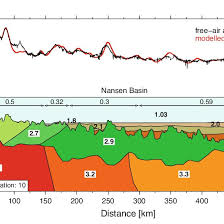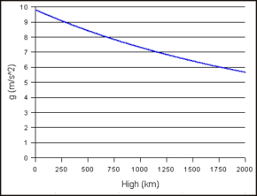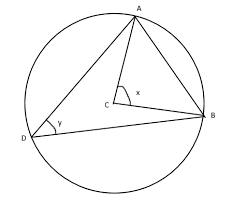## How to Calculate and Solve for Free Air Anomaly | GravityThe image above represents free air anomaly.

To compute for free air anomaly, four essential parameters are needed and these parameters are Observed or Measured Gravity Value (gobs), Reference Value of Gravity (g(λ)), Elevation (h) and Radius of the Earth (R).

The formula for calculating free air anomaly:

δgF = gobs – g(λ) [1 – 2h/R]

Where:
δgF = Free Air Anomaly
gobs = Observed or Measured Gravity Value
g(λ) = Reference Value of Gravity
h = Elevation
R = Radius of the Earth

Let’s solve an example;
Find the free air anomaly when the observed or measured gravity value is 9, reference value of gravity is 10, elevation is 16 and radius of the earth is 4.

This implies that;

gobs = Observed or Measured Gravity Value = 9
g(λ) = Reference Value of Gravity = 10
h = Elevation = 16
R = Radius of the Earth = 4

δgF = gobs – g(λ) [1 – 2h/R]
δgF = 9 – 10 [1 – 2(16)/4]
δgF = 9 – 10 [1 – 32/4]
δgF = 9 – 10 [1 – 8]
δgF = 9 – 10 [-7]
δgF = 9 – -70
δgF = 79

Therefore, the free air anomaly is 79 Gal.

## How to Calculate and Solve for Gravitational Acceleration | GravityThe image above represents gravitational acceleration.

To compute for gravitational acceleration, three essential parameters are needed and these parameters are Gravitational Constant (G), Mass of the Earth (mEand Radius of the Earth (R).

The formula for calculating gravitational acceleration:

a = GmE /

Where:

a = Gravitational Acceleration
G = Gravitational Constant
mE = Mass of the Earth
R = Radius of the Earth

Let’s solve an example;
Find the Gravitational acceleration when the gravitational constant is 6.67E-11, the mass of the earth is 12 and the radius of the earth is 21.

This implies that;

G = Gravitational Constant = 6.67E-11
mE = Mass of the Earth = 12
R = Radius of the Earth = 21

a = GmE /
a = (6.67e-11)(12) / (21)²
a = 8.004e-10 / 441
a = 1.81e-12

Therefore, the gravitational acceleration is 1.81e-12 m/s.

## How to Calculate and Solve for Angle Subtended at the Centre by the Chord | Latitude and LongitudeThe image above represents angle subtended at the centre by the chord.

To compute for angle subtended by the centre at the chord, two essential parameters are needed and these parameters are Length of the Chord of the Small Circle (l) and Radius of the Earth (R).

The formula for calculating the angle subtended by the centre by the chord:

A(2β) = 2sin-1(l2R)

Where:

A(2β) = Angle Subtended at the Centre by the Chord
R = Radius of the Earth
l = Length of the Chord of a Small Circle

Let’s solve an example;
Find the angle subtended at the centre by the chord when the radius of the earth is 6 and the length of the chord of a small circle is 4.

This implies that;

R = Radius of the Earth = 6
l = Length of the Chord of a Small Circle = 4

A(2β) = 2sin-1(l2R)
A(2β) = 2sin-1(42 x 6)
A(2β) = 2sin-1(412)
A(2β) = 2sin-1(0.33)
A(2β) = 2(19.47°)
A(2β) = 38.94

Therefore, the angle subtended at the centre by the chord is 38.94°.